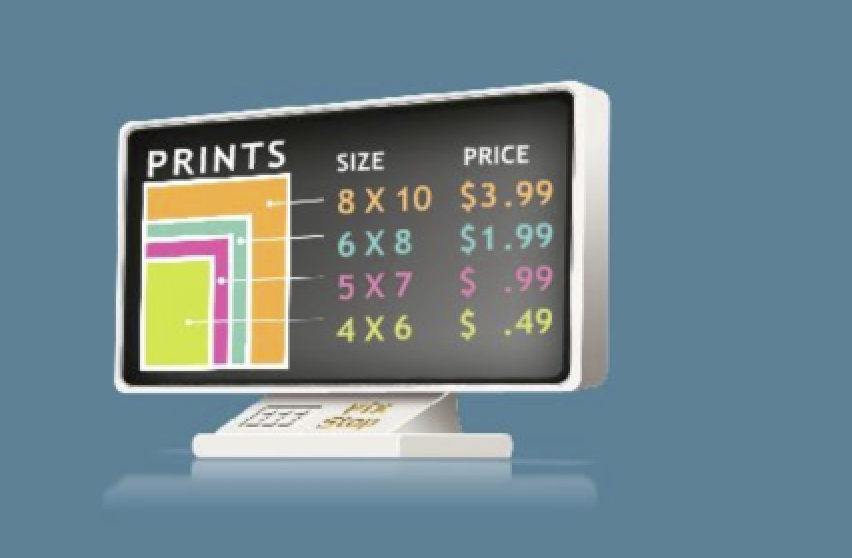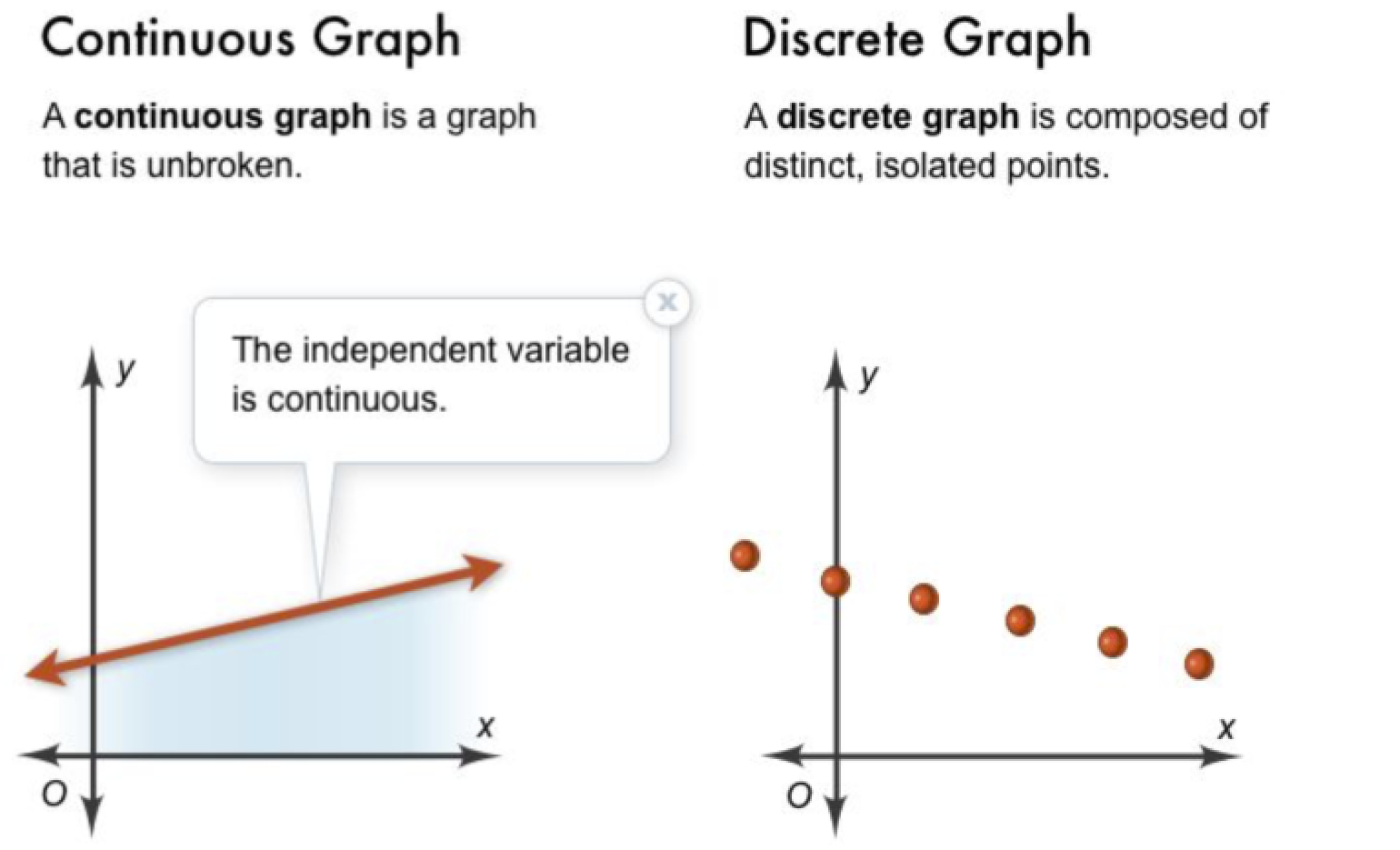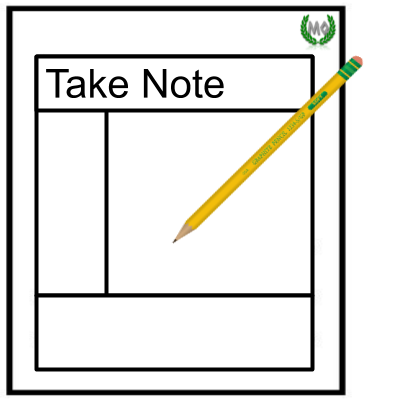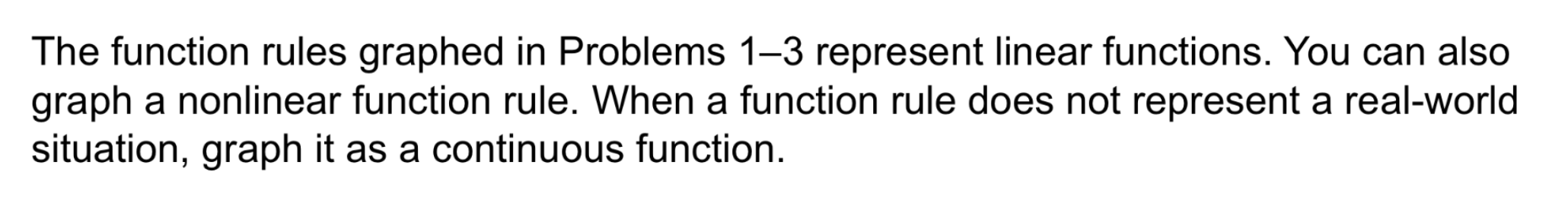Algebra 1 4-4 Guided Practice: Graphing a Function Rule
starstarstarstarstarstarstarstarstarstar
by Matthew Richardson
| 14 Questions1
1
10
Solve It! You are paying to print pictures from your digital camera at the photo shop. You choose one size for all your prints. What is one possible graph of the relationship between the total cost and the number of pictures you print?

Be sure to include relevant graph detail: label axes, indicate units on both axes, and use arrows to represent end behavior, as appropriate.2
10
Problem 1 Got It? What is the graph of the function rule?
Be sure to include relevant graph detail: label axes, indicate units on both axes, and use arrows to represent end behavior, as appropriate.3
4
5
3
10
Take Note: Summarize the process of graphing a function rule.
4
10
Take Note: Graphs of function rules should include specific details. Which of the following are examples of graph detail? Select all that apply.
axis labels on both axes
unit labels on both axes
arrows to represent end behavior of the graph
5
10
Take Note: When is it appropriate to use a graph that only contains one quadrant? You may include an example scenario.
6
10
Problem 2 Got It? The function rule below represents the total weight W, in pounds, of a spa that contains g gallons of water. What is a reasonable graph of the function rule, given that the capacity of the spa is 250 gal?
Be sure to include relevant graph detail: label axes, indicate units on both axes, and use arrows to represent end behavior, as appropriate.7
b
8
Take Note: Some graph detail can be omitted on sketches of graphs. Sketches of graphs can be created quickly and with less precision than standard graphs.
7
10
Take Note: Define continuous graph. Sketch an example of a continuous graph on the canvas.
8
10
Take Note: Define discrete graph. Sketch an example of a discrete graph on the canvas.
9
10
Problem 3 Got It? Graph the function rule.

The amount of water w in a wading pool, in gallons, depends on the amount of time t, in minutes, the wading pool has been filling, as related by the function rule w = 3t.

Be sure to include relevant graph detail: label axes, indicate units on both axes, and use arrows to represent end behavior, as appropriate.
10
5
Problem 3 Got It? Vocabulary: Is the graph for the function rule in the previous item continuous or discrete?
continuous
discrete
11
10
Problem 3 Got It? Graph the function rule.

The cost C for baseball tickets, in dollars, depends on the number n of tickets bought, as related by the function rule C = 16n.

Be sure to include relevant graph detail: label axes, indicate units on both axes, and use arrows to represent end behavior, as appropriate.
12
5
Problem 3 Got It? Vocabulary: Is the graph for the function rule in the previous item continuous or discrete?
continuous
discrete13
20
Problem 4 Got It? What is the graph of the function rule?
Be sure to include relevant graph detail: label axes, indicate units on both axes, and use arrows to represent end behavior, as appropriate.14
14
10
Take Note: Summarize the mathematical content of this lesson. What topics, ideas, and vocabulary were introduced?